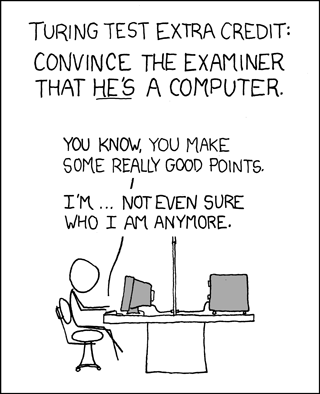Hi All,

As I'm finalizing the CSS conversion of the Math design, I need your help on the images for the 404/Captcha/error pages. You can see the ones I used on the other StackExhange sites.

The idea is to keep it related to the site's topic, but also a bit light-hearted. For example, for Gaming site: Captcha404For Cooking site:

404ErrorYou can append /error, /404, /captcha to see the images from the other newly graduated Stack Exchange sites.

So, what should I use for the Math site? I think it'd be clever to use some in-joke type of math comic strips, assuming they're ok for us to use copy-right wise.

• I think using the "does not exist" symbol ($\not\exists$) for the 404 page would either be really neat or really lame, but I can't tell which. (It would certainly be very hard to make a pretty image out of, which is why I'm not posting this as a serious suggestion.)
– user856
Oct 25, 2010 at 3:59
• @Rahul, it doesn't have to be "pretty." The goal is for the users of the site to "get it." An inside joke if you will. I think your suggestion is great.
– Jin
Oct 25, 2010 at 4:01
• For errors, probably the usual suspects e.g. "you divided by zero!"? For the CAPTCHA, display a calculus problem first and then "just kidding, here's the actual CAPTCHA..." Oct 25, 2010 at 6:06
• @J.M. that's pretty clever! can you find a complex calculus formula? maybe i'll put it on blackboard graphic. I think it'd be fitting, since I'm removing the blackboard treatment from the badges.
– Jin
Oct 25, 2010 at 6:20
• I have quite the number of beasts in my stable, and I'll have to look over them to see what's appropriate @Jin. In the meantime, I believe the others have their own monsters they might like to see! (I was thinking of this replacing the "hello fellow robot" image one sees in the CAPTCHA). Oct 25, 2010 at 6:35
• Now I think about something like Venn diagrams. The set of MSE pages and showing that you are out of it. Some kind of figure is better than a symbol, more interesting to view. BTW look at cstheory.stackexchange.com/404 Jun 8, 2012 at 11:01

Following Jin's encouragement, I'm posting my comment as an answer so people can vote on it.

For the 404 page: The "does not exist" symbol. $$\LARGE\unicode{x2204}$$

• Wouldn't $\neg\exists$ be better?
– user23211
Jun 8, 2012 at 16:53

How about the slogan: "404 is not a very interesting number"

referring to the Ramanujan-Hardy taxi story.

Maybe this XKCD comic for the captcha?Or maybe it's not math-related enough.

Mathematical fallacy for 500 (/error)? For example,

\begin{align} a &= b \\\\ a^2 &= ab \\\\ a^2 - b^2 &= ab - b^2 \\\\ (a+b)(a-b) &= b(a-b) \\\\ a+b &= b \\\\ a+a &= a \quad (∵ a=b) \\\\ 2a &= a \\\\ 2 &= 1\quad?! \end{align}

References:

There might be a rival for $\nexists$, namely the empty set:

$$\LARGE\varnothing$$

Now I wonder if ⊥ was considered for 404 at theoretical computer science.

• I really hope not. Jun 10, 2012 at 15:59

In the text field below, give a proof that for integers $n \ge 3$, there do not exist integers $x,y,z$ with $x^n + y^n = z^n$.

• Fermat's Last Theorem? shouldn't it be n>=2 ? Jun 8, 2012 at 19:01
• @Aarthi Recall the $(3,4,5)$ triangle and other Pythagorean triples. One should also exclude trivial solutions such as $(0,0,0)$ and $(1,0,1)$. Jun 8, 2012 at 19:16
• Ah ha. I stand corrected, which is totally understandable. :D Jun 8, 2012 at 19:18
• HaHA, that would be the best CAPTCHA ever..
– user249332
Feb 2, 2016 at 21:28

May be something about "Divide by zero" for the error page?